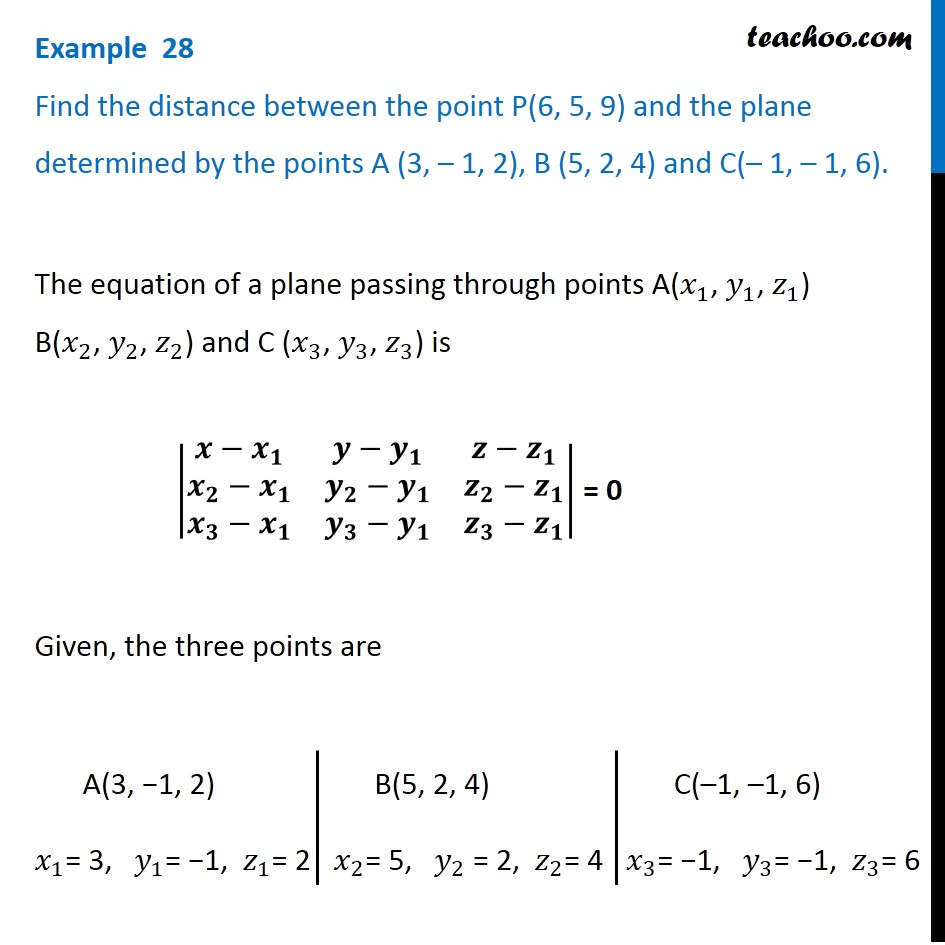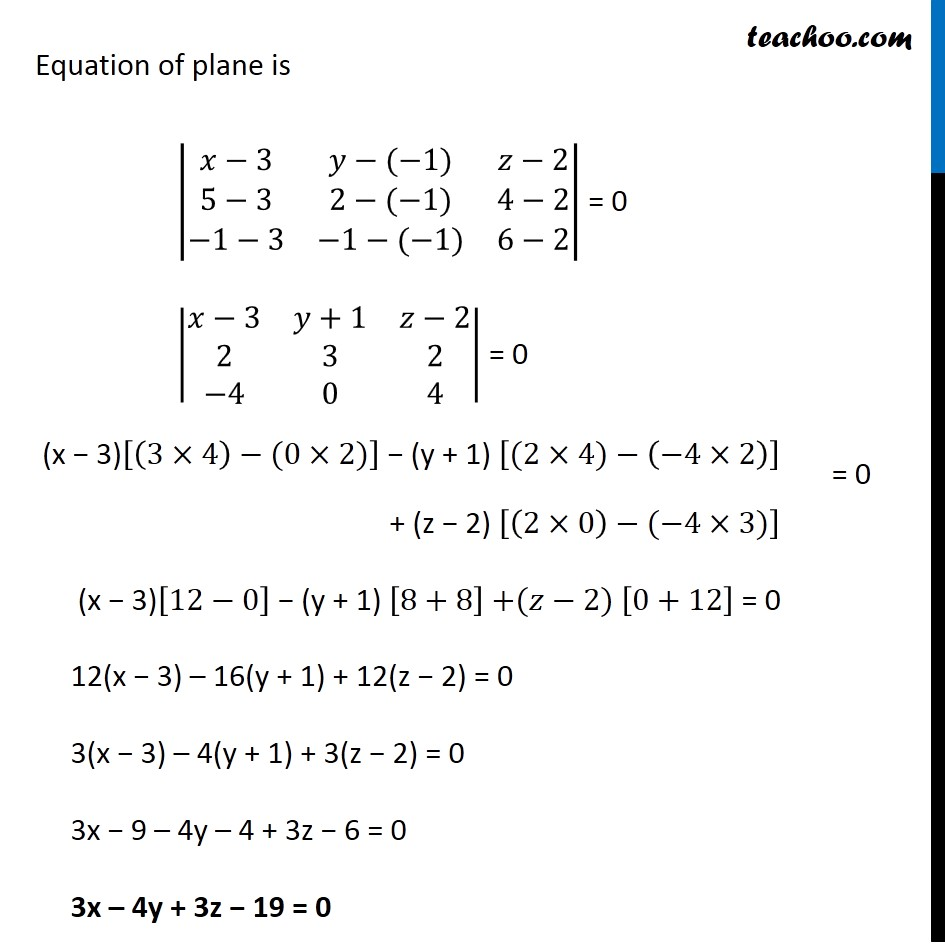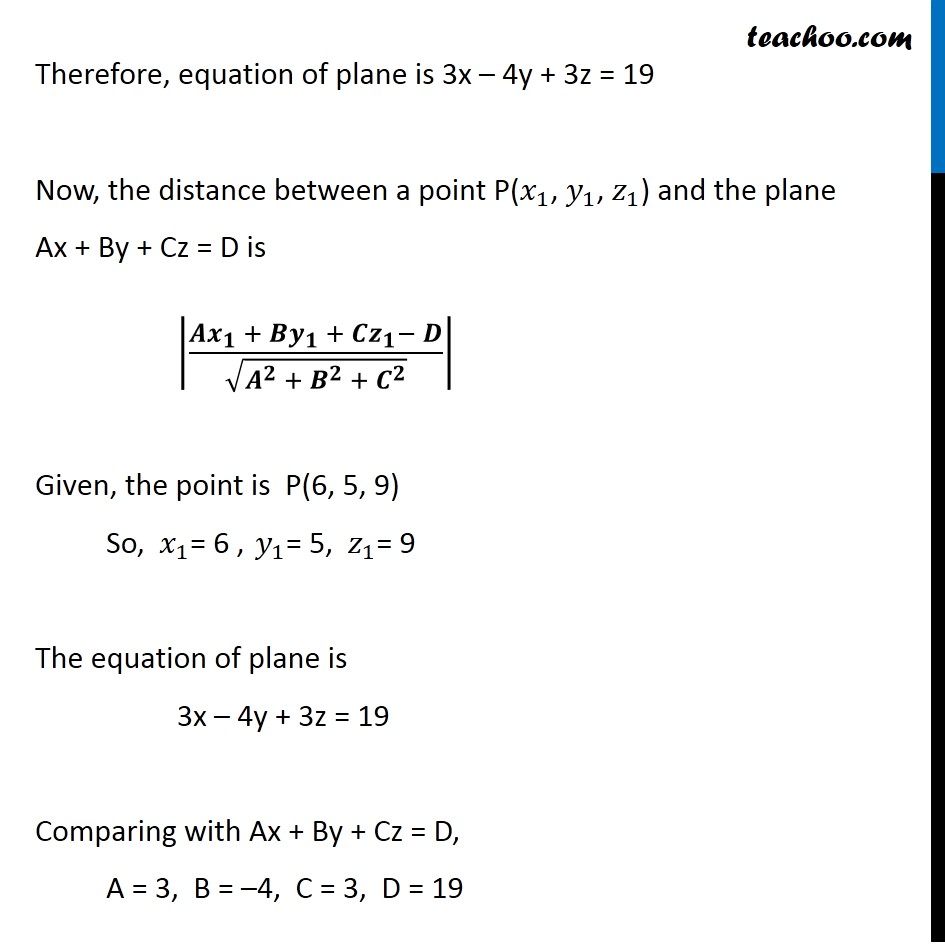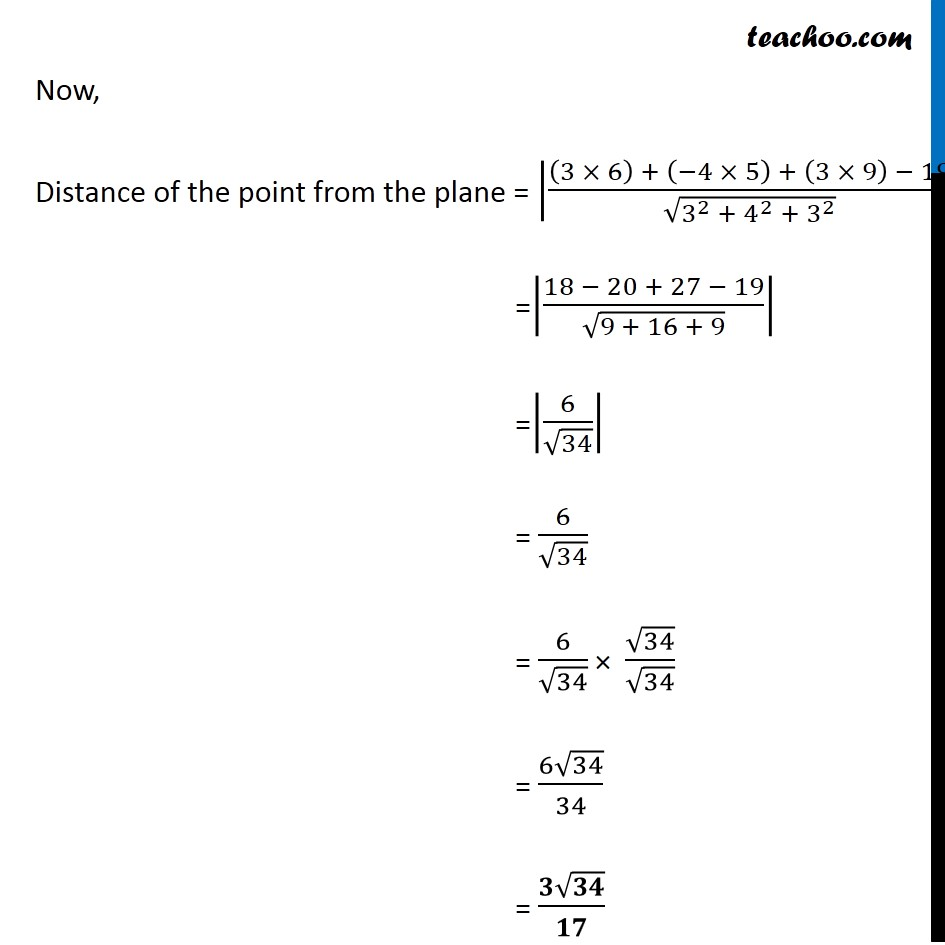Examples

Chapter 11 Class 12 Three Dimensional Geometry
Serial order wiseLearn in your speed, with individual attention - Teachoo Maths 1-on-1 Class

### Transcript

Question 18 Find the distance between the point P(6, 5, 9) and the plane determined by the points A (3, – 1, 2), B (5, 2, 4) and C(– 1, – 1, 6).The equation of a plane passing through points A(𝑥_1, 𝑦_1, 𝑧_1) B(𝑥_2, 𝑦_2, 𝑧_2) and C (𝑥_3, 𝑦_3, 𝑧_3) is |■8(𝒙−𝒙_𝟏&𝒚−𝒚_𝟏&𝒛−𝒛_𝟏@𝒙_𝟐−𝒙_𝟏&𝒚_𝟐−𝒚_𝟏&𝒛_𝟐−𝒛_𝟏@𝒙_𝟑−𝒙_𝟏&𝒚_𝟑−𝒚_𝟏&𝒛_𝟑−𝒛_𝟏 )| = 0 Given, the three points are A(3, −1, 2) 𝑥_1= 3, 𝑦_1= −1, 𝑧_1= 2 B(5, 2, 4) 𝑥_2= 5, 𝑦_2 = 2, 𝑧_2= 4 C(–1, –1, 6) 𝑥_3= −1, 𝑦_3= −1, 𝑧_3= 6 Equation of plane is |■8(𝑥−3&𝑦−(−1)&𝑧−[email protected]−3&2−(−1)&4−2@−1−3&−1−(−1)&6−2)| = 0 |■8(𝑥−3&𝑦+1&𝑧−[email protected]&3&2@−4&0&4)| = 0 (x − 3)[(3×4)−(0×2)] − (y + 1) [(2×4)−(−4×2)] + (z − 2) [(2×0)−(−4×3)] (x − 3)[12−0] − (y + 1) [8+8] +(𝑧−2) [0+12] = 0 12(x − 3) – 16(y + 1) + 12(z − 2) = 0 3(x − 3) – 4(y + 1) + 3(z − 2) = 0 3x − 9 – 4y – 4 + 3z − 6 = 0 3x – 4y + 3z − 19 = 0 Therefore, equation of plane is 3x – 4y + 3z = 19 Now, the distance between a point P(𝑥_1, 𝑦_1, 𝑧_1) and the plane Ax + By + Cz = D is |(𝑨𝒙_𝟏 + 𝑩𝒚_𝟏 + 𝑪𝒛_𝟏− 𝑫)/√(𝑨^𝟐 + 𝑩^𝟐 + 𝑪^𝟐 )| Given, the point is P(6, 5, 9) So, 𝑥_1= 6 , 𝑦_1= 5, 𝑧_1= 9 The equation of plane is 3x – 4y + 3z = 19 Comparing with Ax + By + Cz = D, A = 3, B = –4, C = 3, D = 19 Now, Distance of the point from the plane = |((3 × 6) + (−4 × 5) + (3 × 9) − 19)/√(3^2 + 4^2 + 3^2 )| =|(18 − 20 + 27 − 19)/√(9 + 16 + 9)| =|6/√34| = 6/√34 = 6/√34 × √34/√34 = (6√34)/34 = (𝟑√𝟑𝟒)/𝟏𝟕